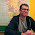## mercoledì 16 maggio 2007

### How can I turn a string into a variable?

If you have

`varname <- c("a", "b", "d")`

you can do

`get(varname) + 2`

for a + 2

or

`assign(varname, 2 + 2)`

for a <- 2 + 2

or

`eval(substitute(lm(y ~ x + variable), list(variable = as.name(varname))`

for lm(y ~ x + a)

At least in the first two cases it is often easier to just use a list, and then you can easily index it by name

`vars <- list(a = 1:10, b = rnorm(100), d = LETTERS) vars[["a"]]`

without any of this messing about.

#### 2 commenti:

1.how can I assign NULL to a variable and have it evaluated properly

for example I want to automate the following two commands
qplot(x,data=myData,weight=depth)
qplot(x,data=myData,weight=NULL)

but use a myWeight variable each time

2.Is the following what you are looking for?

myWeight <- c(12,"NULL")
qplot(x, data=myData, weight=myWeight)
qplot(x, data=myData, weight=myWeight)

HTH